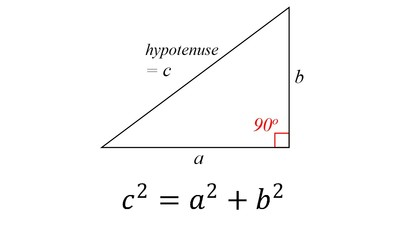# Math History

## Click on any option to know the CORRECT ANSWERS

 Question 6
3/4 is equivalent to which percent?
 A 25% B 50% C 60% D 75%

 Question 7
What mathematician is known for the x/y coordinate plane?
 A Rene Descartes B Pythagoras C Stephen Hawking D Euclid

 Question 8
This formula is known as the ...A Pythagorean Theorem B Quadratic Formula C Distance Formula D Work-force Formula

 Question 9
What 'A' is a formula containing letters and numbers?
 A Algebra B Area C perimeter